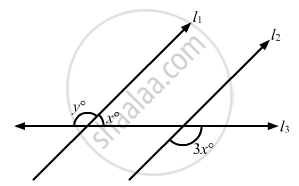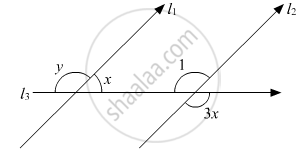# In the Given Figure, If L1 || L2, What is the Value of Y? - Mathematics

MCQ

In the given figure, if l1 || l2, what is the value of y?• 100°

• 120°

• 135°

•  150°

#### Solution

Given figure is as follows:It is given that l1 || l2.

∠1 and 3x are vertically opposite angles, which must be equal, that is,

∠1 = 3x (i)

Also,  ∠1and x are consecutive interior angles.

Theorem states: If a transversal intersects two parallel lines, then each pair of consecutive interior angles are supplementary.

Thus,

∠1 + x = 180°

From equation (i), we get:

3x + x = 180°

4x = 180°

 x = 180°/4

x = 45°

x and y form a linear pair. Therefore, their sum must be supplementary.

Thus,

y + x =180°

Substituting, x = 45° in equation above, we get:

y + 45° = 180°

y = 180° - 45°

y = 135°

Concept: Parallel Lines and a Transversal
Is there an error in this question or solution?
Chapter 10: Lines and Angles - Exercise 10.6 [Page 54]

#### APPEARS IN

RD Sharma Mathematics for Class 9
Chapter 10 Lines and Angles
Exercise 10.6 | Q 16 | Page 54

Share